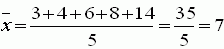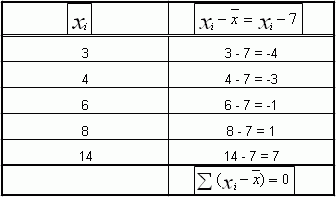Email us to get an instant 20% discount on highly effective K-12 Math & English kwizNET Programs!

#### Online Quiz (WorksheetABCD)

Questions Per Quiz = 2 4 6 8 10

### Middle/High School Algebra, Geometry, and Statistics (AGS)9.21 Properties of Arithmetic Mean

 Some properties of Arithmetic mean If x 1 and x2 are the means of the two groups computed from the values n1 and n2 then the mean x is given by the formula x = n1x1+n2x2/ n1+n2 If each observation in the data is replaced by x, the sum total of all the observations remains unchanged. x = x1,x2,x3,x4........,xn/n So x1,x2,x3,x4........,xn = nx Replacing each observation by x, we get x+x+x........+x = nx If every value of the variable x is either increased, decreased, divided or multiplied by a constant, the observations so obtained also increases, decreases, gets multiplied or gets divided respectively by the same constant. Algebraic sum of the deviation of a set of values from their arithmetic mean is 0 Example Find the sum of the deviations of the variate values 3,4,6,8,14 from their mean. Solution Mean of 3,4,6,8,14 isHence the sum of the deviations about mean = 0 Merits of arithmetic mean 1.) It is rigidly defined. 2.) It is based on all the values. 3.) It is more stable than any other average. Demerits of arithmetic mean 1.) It is highly affected by abnormal values. 2.) The loss of even a single observation makes it impossible to compute the aritmetic mean correctly.
 Q 1: The mean of 10 observations is 20 and that of another 15 observations is 16. Find the mean of all 25 observations.15.717.616.518.2 Q 2: The mean of 5 numbers is 27. If one of the numbers is excluded, the mean gets reduced by 2. Determine the excluded number. 23473568 Q 3: The average grade of all students in class A is 68.4 and in class B is 71.2. If the average of both the classes combined is 70, find the ratio of the number of students in class A to that in class B4:55:63:43:5 Q 4: The algebraic sum of the deviations of 20 observations measured from 30 is 2. Therefore the mean of this observation is ......30.126.340.425.7 Q 5: The mean of 11 numbers is 23. If 5 is added to the new number, find the new mean.28113345 Q 6: The mean of 13 observations is 14. If the mean of the first seven observations is 12 and of the last seven observations is 16, find the seventh observation. 2371421 Question 7: This question is available to subscribers only! Question 8: This question is available to subscribers only!